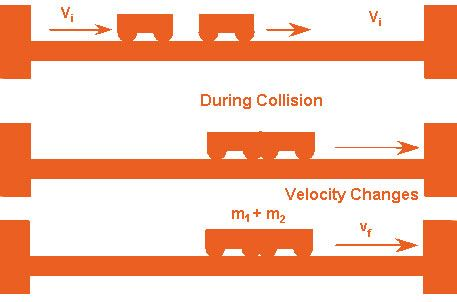# Inelastic Collision Formula

The crash in which kinetic energy of the system is not conserved but the momentum is conserved, then that collision is termed as Inelastic Collision.## Formula of Inelastic Collision

The inelastic collision formula is articulated as

$$\begin{array}{l}m_1u_1 + m_2u_2 = (m_1+m_2)v\end{array}$$

Where
mass of body 1 = m1

mass of body 2 = m2
The initial velocity of body 1 = u1
The initial velocity of body 2 = u2
The final velocity of both the bodies = v

The final velocity for Inelastic collision is articulated as

$$\begin{array}{l}v = \frac{m_1u_1+m_2u_2}{m_1+m_2}\end{array}$$

The inelastic collision formula is made use of to find the velocity and mass related to the inelastic collision.

### Inelastic Collision Solved Example

Problem 1: Compute the final velocity if an object of mass 2 Kg with initial velocity 3 ms-1 hits another object of mass 3 Kg at rest? (Collision is inelastic)
Known:
m1 (Mass)= 2 Kg,
m2(Mass) = 3 Kg
u1 (Initial Velocity of first mass)= 3 ms-1
u2 (Initial velocity of second mass) = 0

$$\begin{array}{l}\text{The Final velocity is given by}\ v = \frac{m_1u_1+m_2u_2}{m_1+m_2}\end{array}$$
$$\begin{array}{l}=\frac{2\times 3+0}{2+3}\end{array}$$

v = 6/5

v = 1.2 m/s